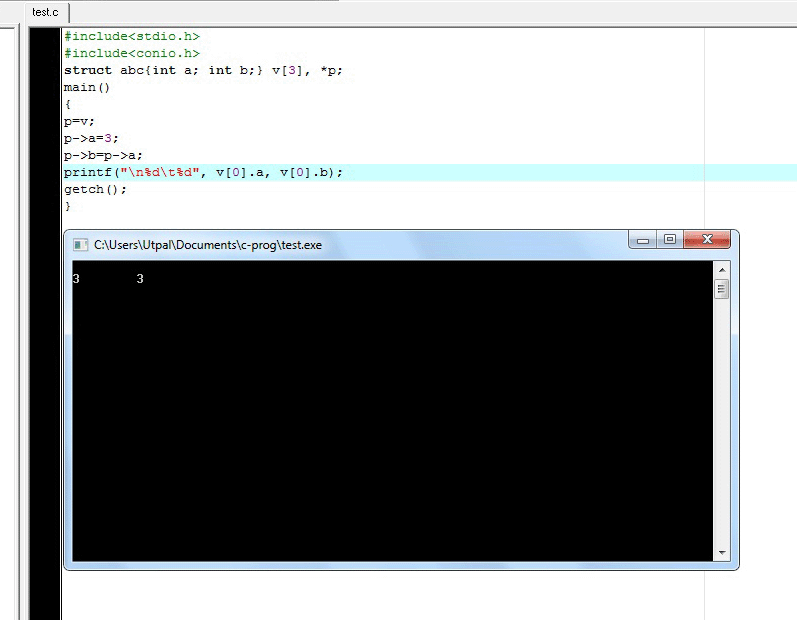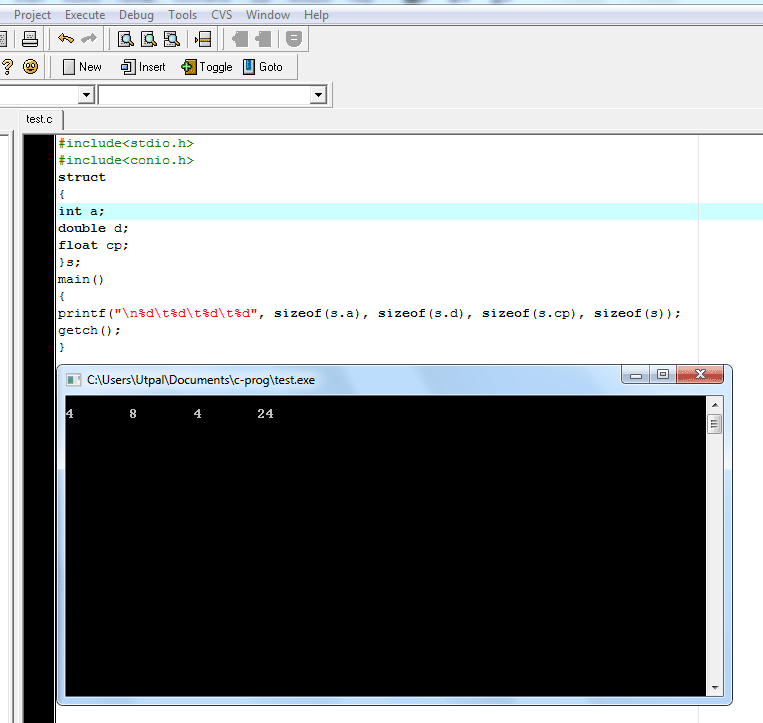# Structures and Unions - C programming question bank with answers in multiple choice type

Questions
1 Size of the following union (assume size of int=2, size of float=4 and size of char=1);

```	union ABC
{
int a;
float b;
char c;
};
```
A 2
B 4
C 1
D 7

union is a data type in which all the members are stored in the same location. The memory size of union is equal to the memory size of the highest member variable. Each members of union can be accessed one by one. In this case size of float is 4. So the size of the union is 4.

2 What is wrong with the following code?

```	struct Person{
char *name;
struct Person Mother, Father;
}Anita;
```
A The ; should appear after the } and Anita be defined later
B name should be defined as an array
C struct Person Mother, Father; must be defined as struct Person *Mother, *Father;
D There is no error in the code

struct is new data type which contains many different types of member variables under a single name.

3 What will be the output of the following code?

```	struct abc{int a; int b;} v, *p;
main()
{
p=v;
p->a=3;
p->b=p->a;
printf("\n%d\t%d", v.a, v.b);
}
```
A 3 4
B 4 3
C Any garbage value
D 3 34 What will be the output of the following program?

```	main()
{
struct emp
{
char name;
int age;
float sal;
};
struct emp e={"Tiger"};
printf("\n%d%f"),e.age, e.sal;
}
```
A 0 0.000000
B Garbage values
C Error
D None of the above5 What will be the output of the following code?

```	struct
{
int a;
double d;
float cp;
}s;
void main()
{
printf("%d\t%d\t%d\t%d", sizeof(s.a), sizeof(s.d), sizeof(s.cp), sizeof(s));
}
```
A 4, 8, 4, 24
B 8, 2, 4, 12
C 10, 4, 6, 4
D 4, 8, 4, 14### Chapters